# Data Interpretation

## Introduction

Data are facts or statistics that are collected from an event. This data, by itself, may not mean anything. This is where the importance of data interpretation arrives. To gather information and analyze these data, we will have to go through data interpretation. We first represent the data in standard forms like a line graph, bar chart, pie chart, and tables. Let's talk about each of them in detail.

Are you preparing Aptitude for your next interview? Check out our Aptitude Preparation guided path.

## Pie Chart

In pie charts, data are shown in circles. The data is grouped into many categories. The circle is divided into many segments or slices, where each segment represents a category. Each segment represents a certain percentage of the total.  The pie chart is always represented as a circle, so 100% of the pie chart is represented in a total of 360°. 1% can be shown as 3.6° in the pie chart.

#### Example

Let us take an example to understand how to read a pie chart. The following pie chart shows the distribution of car sales of 6 car companies.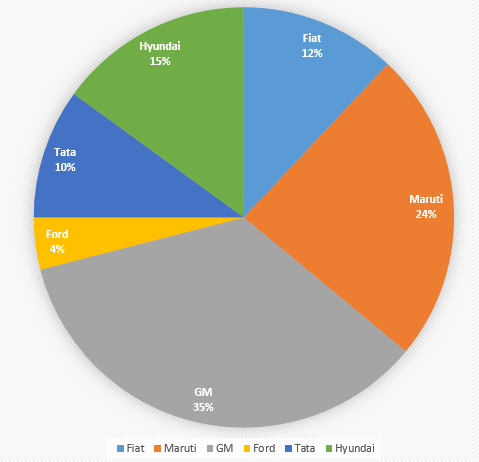Pie chart showing the percentage of car sales of each of the six companies

By seeing this pie chart, we can understand that there are six companies: GM, Fiat, Maruti, Ford, Tata, and Hyundai. This pie chart shows the percentage of car sales of each of the six companies. The pie chart shows that GM (35%) has the highest market share while Ford (4%) has the least market share. Fiat, Maruti, Tata, and Hyundai has 12%, 24%, 10%, and 15% of the total market share, respectively.

Practice a question based on the Pie Chart on CodeStudio.

## Bar Chart

In bar charts, data are presented as rectangular bars with their height or length proportional to their actual values. Here data are variables that can either be discrete or continuous.

There are three types of bar charts, they are:

### Simple Bar Chart

Data interpretation can be quickly made using simple bar charts. It is an easily understandable bar chart having one continuous variable and one discrete variable.

Simple bar graphs are made with the help of two axes- the x-axis and the y axis. The discrete or fixed variables are represented on the x-axis. The y axis represents the continuous variable or the magnitude of that static variable.

#### Example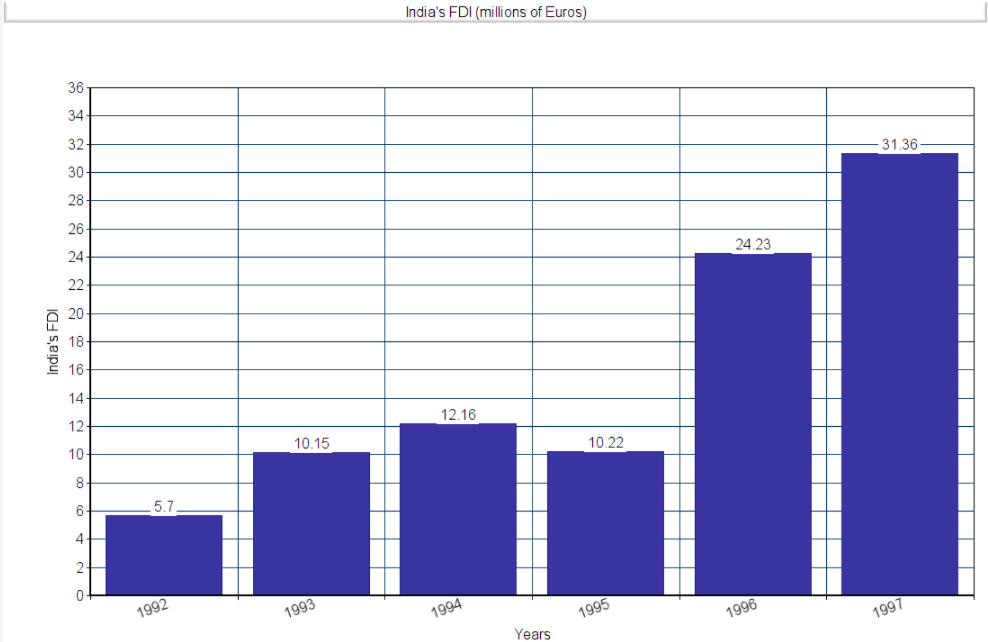Simple bar chart showing India's FDI from 1992 to 1997

To understand and interpret this data, we have to know the variables involved. Here, the year is a discrete variable, whereas India's FDI is a continuous variable.

From the graph, we can understand that in the year 1992, India's FDI was 5.7 million euros. In the year 1997, India's FDI was 31.36 million euros. Hence, by reading this graph, we can see India's FDI from 1992 to 1997.

### Composite Bar Chart

Unlike simple bar charts, composite bar charts have two or more continuous variables. In a composite bar chart, we can read two or more pieces of information about the same discrete variable.

###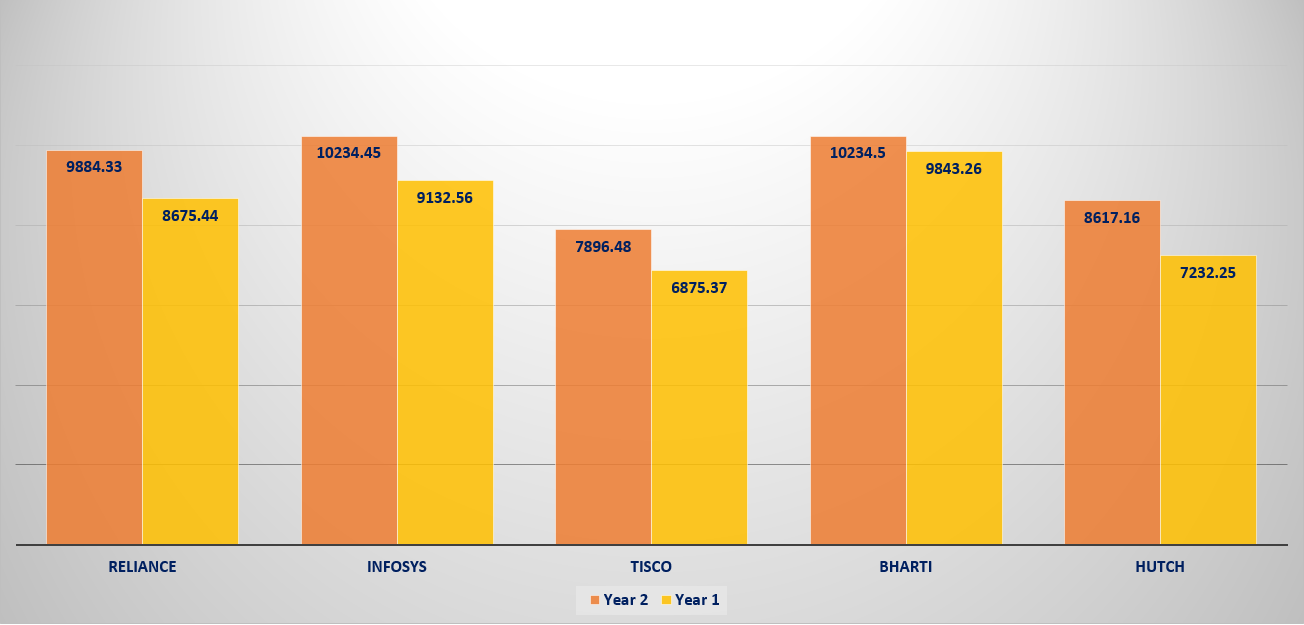Composite bar chart showing the turnover of five companies during two years

Here, the variables involved are a discrete variable Year, company names can be considered discrete variables, and the sales turnover, which is a continuous variable.

Looking at the composite bar chart, we can simultaneously compare two years' data and two or more groups. For instance, for Infosys, the sales turnover in Year 1 is 10234.45 crores, and in Year 2, the sales turnover was 9132.56 crores.

### Stacked Bar Chart

Stacked bar charts can represent multiple continuous variables. We could also break up these continuous variables. A bar represents a whole in stacked bar charts, and the segments represent different parts of that whole. Here each segment is placed on top of the previous segment to create a whole.

#### Example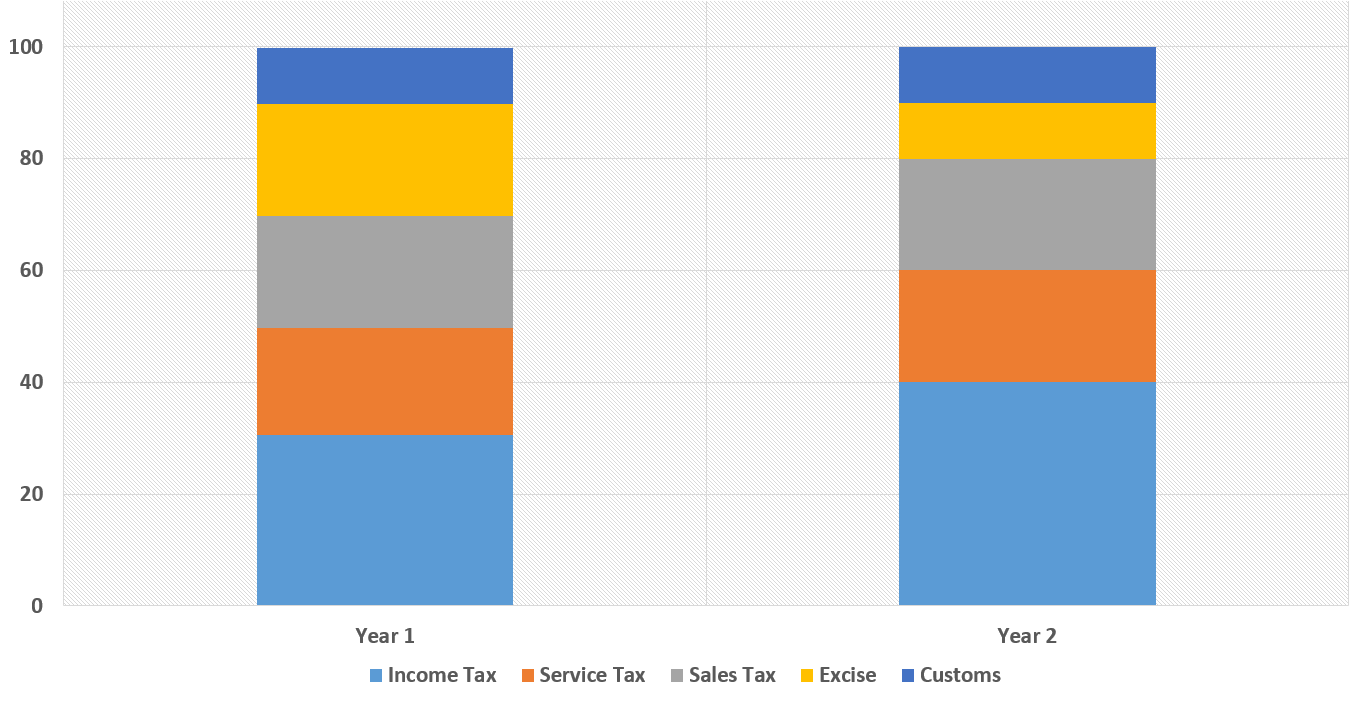Stacked bar chart showing the tax breakdown for two years

Here, the variables involved are a discrete variable year, a discrete variable percentage, and five continuous variables of Taxes- Income tax, Service tax, Sales tax, Excise, and Customs. The different types of taxes are defined as a breakdown for Year 1 and Year 2.

Consider year1; the light blue bar starts from 0 and ends between 20 and 40, so the value is 30-0=30. The red color bar starts from the middle of 20 and 40, which is 30, and ends at 50(middle of 40 and 60), so the value of the red bar is 50-30=20. The grey bar starts at 50 and ends at 70, so the value is 70-50=20. The orange bar starts at 70 and ends at 90, so the value is 90-70=20. Finally, the dark blue bar starts at 90 and ends at 100, so the value is 100-90=10. In this way, we can get the values of each bar in Year 2 also.

Practice a question based on the Bar Chart on CodeStudio.

## Line Chart

Line charts are also called X-Y charts. In line charts, the discrete variables are plotted against the continuous variables. Line charts or line graphs are a very frequently used data interpretation method. These charts are helpful to determine rates of change, trends and show comparisons concerning some time series.

### Line Chart With Single Continuous Variable

We can plot a line chart with a single continuous variable. Here one continuous variable is plotted against the corresponding discrete variables.

#### Example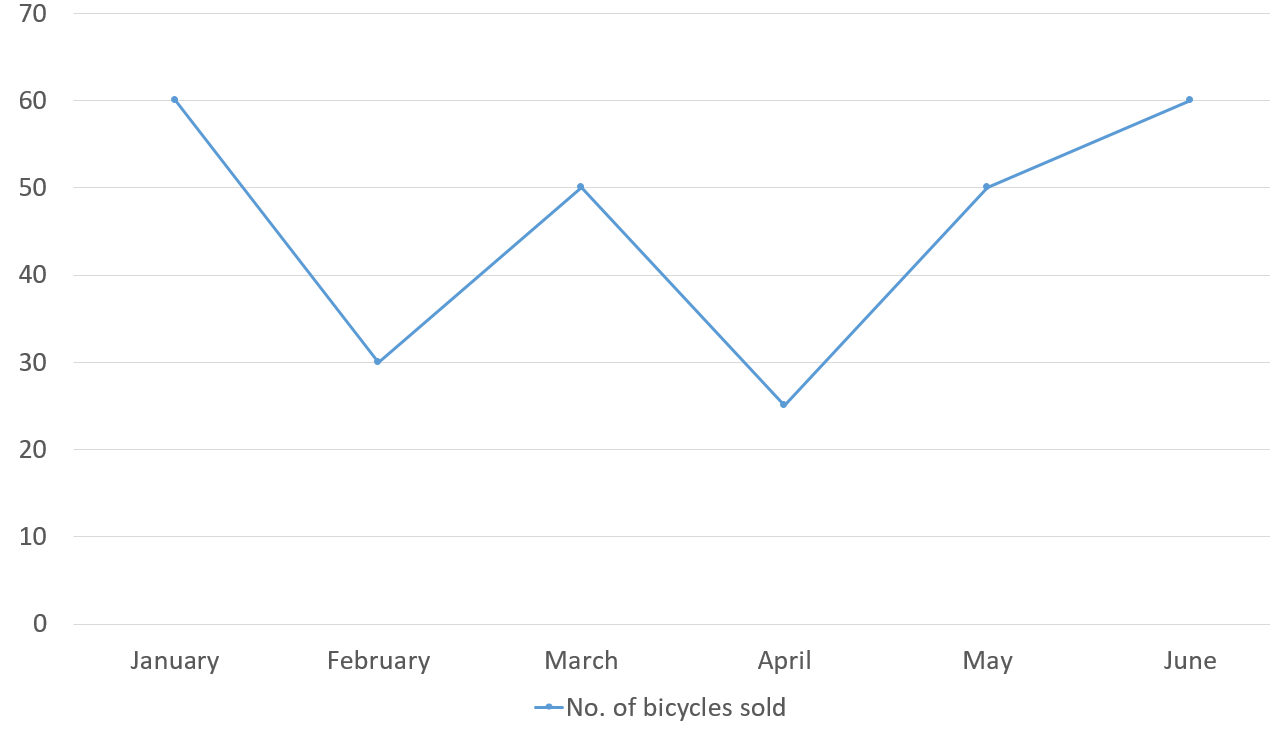Line chart showing the bicycle sales during January - June

This line chart shows the number of bicycles sold by a seller each month. Here the continuous variables are the number of bicycles sold each month, and the discrete variables are the name of months. We can make data interpretation very quickly in this method. For example, we can say that the number of bicycles sold in January is 60 and that of March is 50.

### Line Chart With Multiple Continuous Variables

We can also draw a line chart with multiple continuous variables. Let us go through an example to understand this better.

#### Example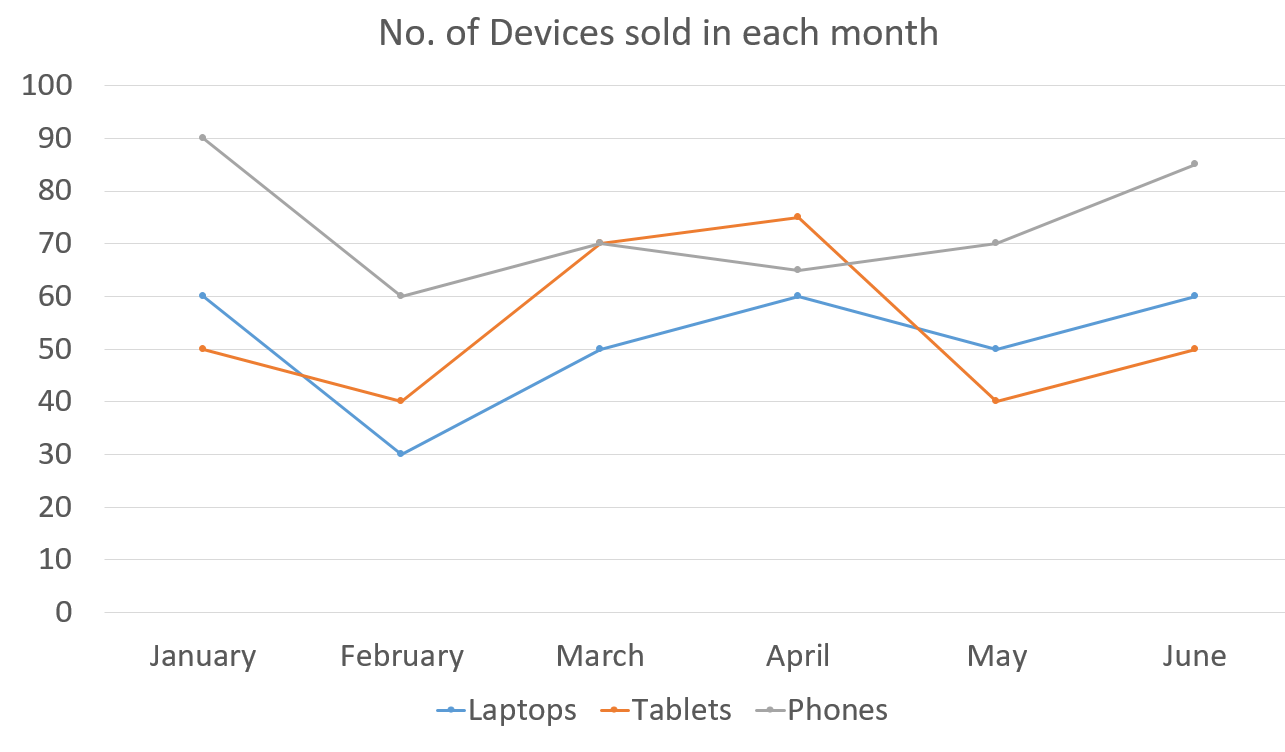Line chart showing the sales of various devices during January - June

This chart shows the number of devices sold each month. Three types of devices are sold by the seller- Laptops, Phones, and Tablets. By looking at the chart, we can compare the sales turnover of each of these products in a particular month.  In January, 60 laptops, 50 tablets, and 90 phones were sold. In April, the number of Tablets sold by the seller was more than the number of Phones sold. Hence, we could make analysis and data interpretation easily with a line chart.

Practice a question based on the Line Chart on CodeStudio.

## Table

In tables, data is represented in the form of horizontal rows and vertical columns. Tables are comparatively more flexible methods of representing data. These are more adaptable and versatile. Hence this is an effective way of data interpretation. In tables, any number of continuous variables may be used against any number of discrete variables. Data that is represented on any chart could easily be represented on a table.

#### Example

Table showing the Total Literacy, Female Literacy, and Change in % population growth rate in various states

Here, we can understand that this could easily be converted to a composite bar chart. The variables involved in this chart are: discrete variables State, discrete variables Year, and three continuous variables - Total Literacy, Female Literacy, and Change in % population growth rate.

Looking at the table, we can come to many conclusions, such as from 1981 to 1991, the state with the highest female literacy is Kerala. The state with the highest total literacy is Himachal Pradesh. The state with the least total literacy is Bihar. Andhra Pradesh increased its growth rate by 0.09 by the end of 10 years. Hence data interpretation could be made with the help of tables as shown above.

Practice a question based on the Table on CodeStudio.

Q1. What are some of the ways to represent data?

Ans: Some ways to represent data include textual representation, diagrammatic representation, and tabular representation.

Q2. What are the different types of bar charts?

Ans:- Some bar charts include simple bar charts, composite bar charts, and stacked bar charts.

Q3. What is a pie chart?

Ans: A pie chart is a circular statistical graphic divided into slices to illustrate numerical proportion.

## Key Takeaways

Data interpretation is the knowledge that is essential to us in the modern world. Data can be collected and stored as Pie Charts, Line Charts, Bar Graphs, and Tables. To analyze these data, we must know how to interpret them correctly. This is where data interpretation comes into play. The data that had been collected can be represented in any one of the forms mentioned above. These data are then analyzed and reviewed according to our needs. Then we come to relevant conclusions based on our analysis. Pie charts, Line charts, Bar charts, and tables are widely used data interpretation methods to analyze the collected data.